# 8 wooden

8 wooden poles are used for pillers and length of the pillers are from an arithemetric progression if the second pole is 2 metre and the sixth pole in order 5 meters, find the different between the sixth pole and seventh pole.

d =  0.75 m

### Step-by-step explanation: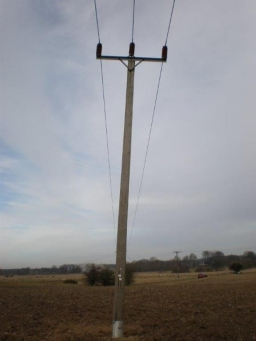Did you find an error or inaccuracy? Feel free to write us. Thank you!## Related math problems and questions:

• Arithmetic progression 2The 3rd term of an Arithmetic progression is ten more than the first term, while the fifth term is 15 more than the second term. Find the sum of the 8th and 15th terms of the Arithmetic progression if the 7th term is seven times the first term.
• Geometric progressionIn geometric progression, a1 = 7, q = 5. Find the condition for n to sum first n members is: sn≤217.
• Common difference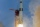The 4th term of an arithmetic progression is 6. If the sum of the 8th and 9th terms is -72, find the common difference.
• Eight blocks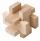Dana had the task to save the eight blocks of these rules: 1. Between two red cubes must be a different color. 2. Between two blue must be two different colors. 3. Between two green must be three different colors. 4. Between two yellow blocks must be four
• The sides 2The sides of a trapezoid are in the ratio 2:5:8:5. The trapezoid’s area is 245. Find the height and the perimeter of the trapezoid.
• What is 10What is the 5th term, if the 8th term is 80 and common ratio r =1/2?
• Find the 13Find the equation of the circle inscribed in the rhombus ABCD where A[1, -2], B[8, -3] and C[9, 4].
• Insert 3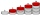Insert five arithmetic progression members between -7 and 3/2.
• Street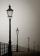Straight streets is long x = 475 meters. At the beginning and at the end of the street are poles. The poles are spaced 25 m. How many poles are on the street?
• Telegraph poles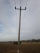The bases of two adjacent telegraph poles have a height difference of 10.5 m. How long do the wires connect the two poles if the slope is 39° 30´?
• The pole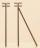The telegraph pole is supported by a 4 m bullet at 3/4 of its height, the end of which is at a distance 2.5 m from the pole post. Calculate the height of the telegraph pole.
• Area of gardenIf the width of the rectangular garden is decreased by 2 meters and its length is increased by 5 meters, the area of the rectangle will be 0.2 ares larger. If the width and the length of the garden will increase by 3 meters, its original size will increas
• Fewer than 500 sheep,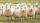There are fewer than 500 sheep, but if they stand in a double, triple, quadruple, five, and sixth-order, one sheep will remain. But they can stand in the seventh order. How many are sheep?
• Negative differenceFind four arithmetic progression members between 7 and -6.
• Find the 2Find the term independent of x in the expansion of (4x3+1/2x)8
• Two geometric progressions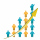Insert several numbers between numbers 6 and 384 so that they form with the given GP numbers and that the following applies: a) the sum of all numbers is 510 And for another GP to apply: b) the sum of entered numbers is -132 (These are two different geome
• Population growth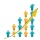How many people will be on Earth from two people for 5,000 years, if every couple has always 4 children, (2 boys and 2 girls) at the age of 25-35, and every man will live 75 years?